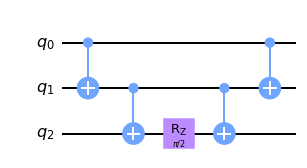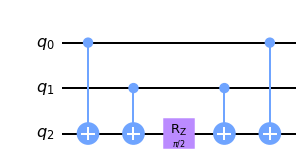# Classiq contest writeup

Recently our engineers took part in a contest organized by the quantum company Classiq as we mentioned in a previous post.

Unlike previous quantum competitions, such as the IBM quantum challenge, Classiq used a different format — they presented the crowd with four different puzzles, each with their own prizes. Below we present our solutions to the puzzles.

### Hamiltonian simulation problem

Let us begin with a few definitions. Assume we fixed a Hilbert space $$\mathcal H$$. By the hamiltonian we mean any operator $$H \colon \mathcal H \rightarrow \mathcal H$$, which is self-conjugate. In our case the underlying Hilbert space is $$\mathbb {C^2} ^ {\otimes 10}$$, that is the space of $$10$$ qubits. The key property of self-conjugate operators is that $$\exp(itH)$$ is a unitary operator, which implements the evolution of the quantum system described by $$H$$ in time $$t$$.

In this challenge we are given a hamiltonian $$H$$ written in a certain form and we are asked to provide a circuit $$C$$, which approximates $$\exp(itH)$$ for $$t=1$$ with a given precision. In other words:

• $$||\exp(iH) - C|| \leq 0.1$$, where $$|| \cdot ||$$ is the spectral norm defined for any matrix $$M$$ as $$\max_{v \in V} \frac {|Mv|}{|v|}$$, where $$|\cdot|$$ is the ordinary Euclidean norm on vectors.
• The depth of $$C$$ must be as small as possible.

A part of the full hamiltonian given in the file input.txt is printed below:

INPUT_PATH = "input.txt"

with open(INPUT_PATH) as file:

print(hamiltonian_string, "\n...")

0.003034656830204855 * IIIYYIIIYY

+  0.003034656830204855 * IIIXXIIIYY

+ 0.003034656830204855 * IIIYYIIIXX

+ 0.003034656830204855 * IIIXXIIIXX

- 0.008373361424264817 * YZZZYIIIYY

...


The above hamiltonian is represented in the basis of the Pauli operators. Any self-conjugate operator acting on $$n$$ qubits can be written uniquely as a real linear combination of operators $$P_1 \otimes \ldots \otimes P_n$$, where $$P_1, \ldots, P_n$$ are the Pauli matrices $$I, X, Y, Z$$. For simplicity we omit the $$\otimes$$ symbol in the above sum.

We use qiskit to read our hamiltonian, as shown below:

from qiskit.opflow import *

from qiskit.quantum_info import Pauli

import numpy as np

with open(filename) as input_file:
lines = [x for x in input_file.readlines() if x != "" and x != "\n"]
lines = "+ " + lines
for line in lines:
sgn, coeff, _, pauli = line.split()
coeff = float(coeff) if sgn == "+" else -float(coeff)
op = PauliOp(Pauli(pauli), coeff)
yield op



To compute the error generated by the solution circuit we need two functions:

def dist(A, B):
return np.linalg.norm(A - B, ord=2)

def su_dist(U, Ul):
λ = np.trace(Ul.conj().T @ U) / U.shape
return dist(λ * Ul, U)


Mind that circuits differing merely by the global phase are considered equal. For this reason we must keep a procedure for comparing them. The target operator $$U$$ constructed by us will be compared with the exact operator:

U = sum(H).exp_i().to_matrix()


### Trimming

The first thing we tried was removing some of the hamiltonian's summands. Intuitively, eliminating those with the smallest coefficients should lead to the smallest error. We must however remember that the more summands we trim, the closer to exact our simulation with the resulting hamiltonian must be. This is to keep the error below the $$0.1$$ value specified in the challenge.

sortedH = sorted(H, key=lambda x: abs(x.coeff))

trimmed_count = 75
trimmedH = sum(sortedH[trimmed_count:])

trimmedU = trimmedH.exp_i().to_matrix()

print(f"distance={dist(U, trimmedU)} < 0.1")

distance=0.07024338072999671 < 0.1


### Trotterization

We will utilize the product formula approach. In its simplest form it is given as

$$\exp\left(t\sum_{i = 0}^k H_i\right) \approx \left(\prod_{i = 0}^k \exp\left(\frac t N H_i \right)\right) ^ N$$

Note that we may choose different orderings of the sequence $$H_0, \ldots, H_k$$ but this can lead to varying results. Ultimately, it is also possible to combine the above product formula with a special case of the second-order formula:

$$\exp\left(H_1 + H_2\right) \approx \exp\left(\frac {H_1} 2\right) \exp\left(H_2\right) \exp\left(\frac {H_1} 2\right)$$

### Implementing primitive gates

$$\newcommand{\ket}{\left|#1\right>}$$

Taking into account everything we already said, it suffices to implement gates of the form $$\exp\left(itP_1\ldots P_k\right)$$, where $$P_i$$ are the Pauli matrices. First we note that

$$\exp(itZ^{\otimes n})\ket{a_1\ldots a_k} = \begin{cases} \ket{n} & \text{if } {a_1 + \ldots + a_k = 0 \mod 2} \\ e^{2it} \ket{n} & \text{otherwise} \end{cases}$$

Qiskit provides two implementations of such a gate based on similar concepts. First using the $$CX$$ gates we store the sum mod $$2$$ of the bits, then we apply the $$Rz(t)$$ gate and finally we uncompute. This can be done either by the fountain or chain structures (see figure below).

Because every Pauli gate can be constructed by conjugating the $$Z$$ gate with some $$1$$-qubit Clifford unitaries, we use the implementation of $$\exp(itZ^{\oplus n})\ket{n}$$ to get every other gate we are interested in. Having that said we may try our first shot.

Chain:Fountain:from qiskit import QuantumCircuit, transpile
from qiskit.synthesis import LieTrotter
from qiskit.circuit.library import PauliEvolutionGate
from qiskit import execute
from qiskit import Aer

def first_try(H, times=10):
suzuki = LieTrotter(reps=1)
Ht = PauliEvolutionGate(H, 1.0)
circuit = suzuki.synthesize(Ht)

circ = transpile(circuit, optimization_level=3, basis_gates=['u3', 'cx'])
print(f"Depth after optimization={circ.depth()}")

res = execute(circ, backend=Aer.get_backend('unitary_simulator'))
Ul = np.asarray(res.result().get_unitary())
print(f" distance={su_dist(U, Ul)}")

first_try(trimmedH)

Depth after optimization=1439
distance=0.09876674736507203


concluding that 'distance' is within the bounds, but 'depth' is large. The latter can be minimized by replacing the implementation of our primitive gate (qiskit's default is chain). Picking 'the fountain' as a trial implementation gives

def second_try(H):
suzuki = LieTrotter(reps=1, cx_structure='fountain')
Ht = PauliEvolutionGate(H, 1.0)
circuit = suzuki.synthesize(Ht)

circ = transpile(circuit, optimization_level=3, basis_gates=['u3', 'cx'])
print(f"Depth after optimization={circ.depth()}")

res = execute(circ, backend=Aer.get_backend('unitary_simulator'))
Ul = np.asarray(res.result().get_unitary())
print(f" distance={su_dist(U, Ul)}")

# first_try(trimmedH)

second_try(trimmedH)

Depth after optimization=993
distance=0.09876674736507288


The above outcome is far better due to the structure of the CX gates allowing for more cancellations. Following up on this promising result, we further optimize the circuit to achieve even more cancellations. In order to do so we note that the qubit on which we put the sum mod $$2$$ can be chosen freely. Hence we can choose these qubits to be the same qubits as before. Although such an approach may sound suboptimal, we have decided to test it:

def make_fountain(pauli: Pauli, w, previous=None):

qc = QuantumCircuit(len(pauli))
nontrivials = [i for i, p in enumerate(pauli) if p != Pauli("I")]
if not nontrivials:
return qc
if previous is not None and previous in nontrivials:
target_qubit = previous
else:
target_qubit = nontrivials
diag = QuantumCircuit(len(pauli))
for i in nontrivials:
if pauli[i] == Pauli('X'):
diag.sdg(i)
if pauli[i] in [Pauli('Y'), Pauli('X')]:
diag.h(i)
qc = qc.compose(diag)
for i in nontrivials:
if i != target_qubit:
qc.cx(i, target_qubit)
qc.rz(w * 2, target_qubit)
for i in nontrivials:
if i != target_qubit:
qc.cx(i, target_qubit)
qc = qc.compose(diag.inverse())
return qc, target_qubit

class FountainMaker:

def __init__(self):
self.history = [None]

def __call__(self, pauli: Pauli, w):
result, target_qubit =  make_fountain(pauli, w, self.history[-1])
self.history.append(target_qubit)
return result

def third_try(H):
suzuki = LieTrotter(reps=1, atomic_evolution=FountainMaker())
Ht = PauliEvolutionGate(H, 1.0)
circuit = suzuki.synthesize(Ht)

circ = transpile(circuit, optimization_level=3, basis_gates=['u3', 'cx'])
print(f"Depth after optimization={circ.depth()}")

res = execute(circ, backend=Aer.get_backend('unitary_simulator'))
Ul = np.asarray(res.result().get_unitary())
print(f" distance={su_dist(U, Ul)}")

third_try(trimmedH[:-1])

Depth after optimization=908
distance=0.0987667473650693


The difference between the exact $$\exp$$ of our trimmed hamiltonian and the simulation is large - around 30 %. To circumvent this problem we simultaneously remove more terms from $$H$$ and decrease the simulation error by using the second-order approximation:

def last_try(H, split_count):
suzuki = LieTrotter(reps=1, atomic_evolution=FountainMaker())
# We select a certain amount of the biggest summands to be split in half
H1t = PauliEvolutionGate(H[split_count:] / 2, 1.0)
H2t = PauliEvolutionGate(H[:split_count], 1)
res1 = suzuki.synthesize(H1t)
res2 = suzuki.synthesize(H2t)

qc1 = res1
qc1 = qc1.compose(res2)
qc1 = qc1.compose(res1)
circ = transpile(qc1, optimization_level=3, basis_gates=['u3', 'cx'])

print(f"Depth after optimization={circ.depth()}")

res = execute(circ, backend=Aer.get_backend('unitary_simulator'))
Ul = np.asarray(res.result().get_unitary())
print(f" distance={su_dist(U, Ul)}")

last_try(trimmedH[35:-1], 155)

Depth after optimization=751
distance=0.09127680220865292


By doing so we have reduced gate depth by almost 50% in comparison to our first attempt. This was enough to achieve 3rd place.